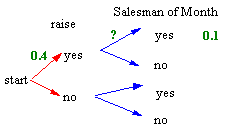### CFA Practice Question

There are 985 practice questions for this topic.

### CFA Practice Question

Sal has a 60% probability of being Salesman of the Month. He has a 40% probability of getting a raise. Sal has a 10% probability of being Salesman of the Month and getting a raise. What is P(Sal gets Salesman of the Month given he gets the raise)?

A. 25%
B. 30%
C. 16.7% to nearest 0.1%

In a tree diagram, the event Salesman of Month given raise is represented by the top branch of the second set of branches. To find the probability of this branch we use: 0.4x = 0.1. Now, x = 0.1/0.4 = 1/4 = 0.25. Thus, P(Sal gets Salesman of the Month given raise) = 25%.